•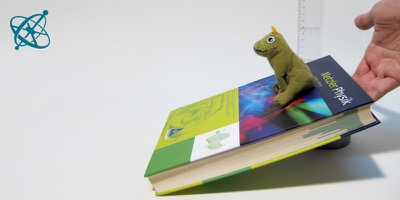A quick way to study friction:

•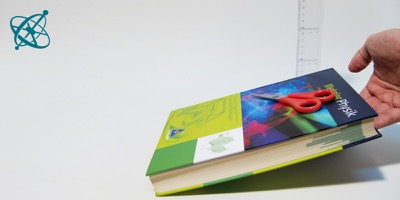Lift a book with the test object on it.

•When does the object start to slide?

•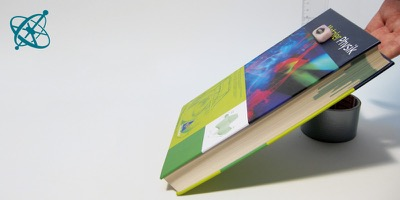Objects with higher friction start sliding at a larger slope,

•while those with a lower friction slide earlier.

•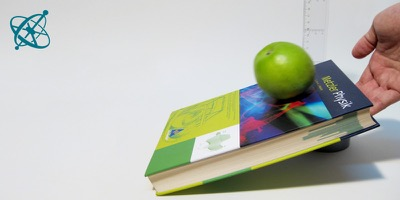But what determines the friction?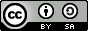www.sciensation.org | Ciênsação hands-on experiments are published as Open Educational resources under a Creative Commons Attribution-ShareAlike 4.0 International License.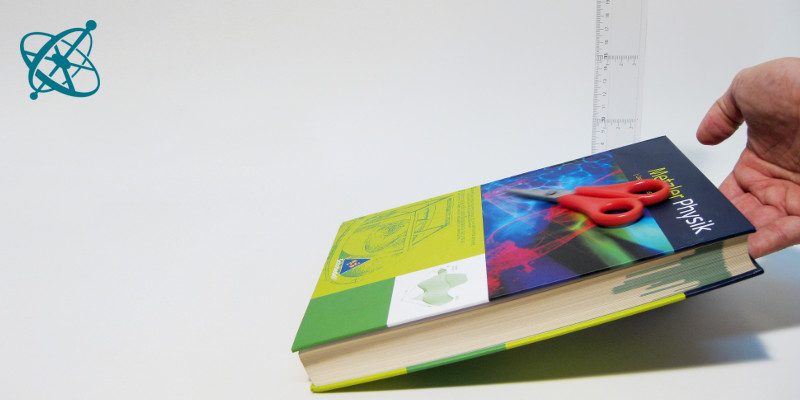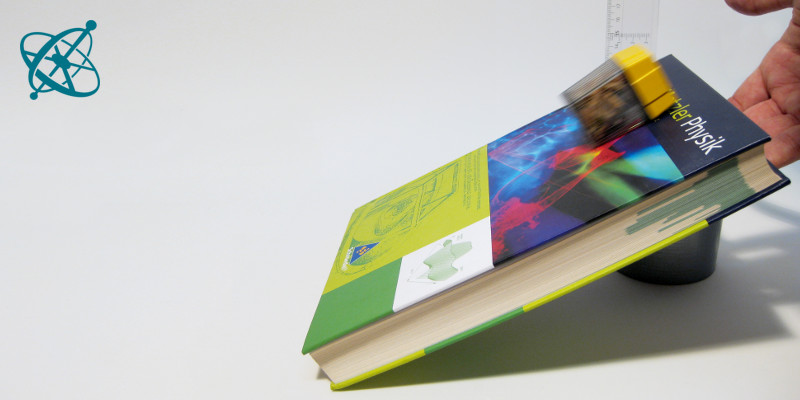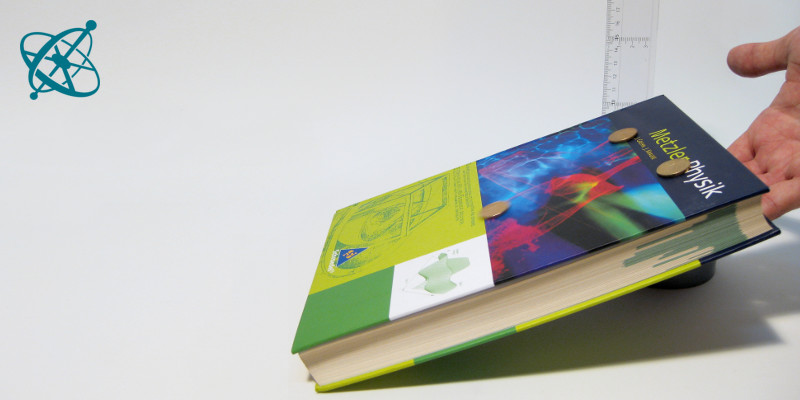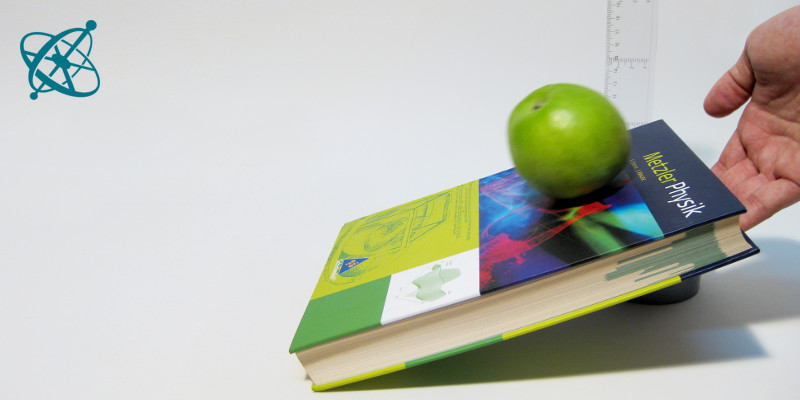Physics
age: 14 – 16

# Sliding

An improvised inclined plane is all it takes to understand the basics about dry static friction.

Learning objective

Dry friction depends on the surface properties and the force pressing surfaces together, but not on the contact area.

Designing experiments that allow to study different factors separately.

Books
Different test objects
Preparation

If your students have no rulers to measure the inclination, they can also attach some paper to another book and mark on it the height at which an object begins to slide.

Place an object on a book or a similar sturdy object with a planar surface. Then slowly lift one edge of the book and measure with a ruler at what rise (and thus what slope) the objects begins to slide. Test different objects on different surfaces.

1. What prevents the objects from immediately sliding down the slope at the smallest inclination?

2. What determents the strength of friction? Can you demonstrate this with an experiment?

Guiding Questions (if needed)

How can you test if surface properties influence friction?
› By comparing two sides of an object that have the same area but are made of different materials. Alternatively one could change a surface by applying sticky tape.

How do you probe the influence of the contact area?
› By comparing two sides of an object that have identical surface properties but different dimensions.

Does friction depend on the contact area?
› No. If the force is distributed over a larger or smaller area makes no difference to the total force pressing the surfaces together.

Does the object's mass and thus weight make any different?
› Not in this experiment. If you stack two objects, they will start sliding at the same angle as a single one.

So does that mean that the force pressing the two surfaces together does not matter?
› No. When the object is pushed against the book, e.g. with a finger, it slides at a larger angle or is even held up.

Findings

The dry friction force depends on the surface properties and the normal force pressing these surfaces together. The contact area, on the other hand, has no influence on the friction (Amonton's first law).

Interestingly, the mass m has no influence on the angle at which an object begins to slide. This angle is reached when the force pointing downward the slope is larger than the friction force:

Fdownward ≥ Ffriction
m g sin(α) ≥ µ m g cos(α)

Since both forces depend equally on the mass, the angle αslide only depends on the friction coefficient µ, which your students can calculate – using their own measurements – as µ = arctan(αslide).

The important aspect of this simple activity is that your students learn to analyze different factors independently. To come to a meaningful conclusion, they have to find ways to vary only one parameter at a time, holding all others constant.

Related Experiments